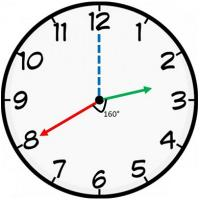# Logical Reasoning for Job Interviews - Set 1

1)   In the following question, choose the correct code form.

The number/word group in the question is to be codified according to the following letter codes:

 Number 9 8 7 6 5 4 3 2 1 0 Letter Q U I C K L Y R O D

RODRICK

a. 2104865
b. 2104765
c. 2102765
d. 2103765
 Answer  Explanation ANSWER: 2102765 Explanation: No explanation is available for this question!

2)   In the following question, choose the correct code form.

If in a certain language, MORALE is coded as 296137, CHARCOAL is coded as 45164913, how is the word REAL-MOLE coded in that language?

a. 67193237
b. 67132937
c. 67123937
d. 67172937

Explanation:

 Letter A M L C H R E O Code 1 2 3 4 5 6 7 9

 Letter R E A L M O L E Code 6 7 1 3 2 9 3 7

3)   1. There are 16 teams divided into 2 pools of 8 each.
2. Each team in a group plays against one another on a round-robin basis.
3. The top four teams from each group will qualify for the next round i.e. round 2.
4. In case of teams having the same number of wins, the team with better run-rate would be ranked ahead.
5. Draws are not allowed.

What is the minimum number of wins required to guarantee qualification in the next round?

a. 4
b. 5
c. 6
d. 7
 Answer  Explanation ANSWER: 6 Explanation: In this case consider the mean of first 5 higher seeded teams (7+6+5+4+3)/5=5 So, it may be the case that 5 teams can end up having 5 wins each. And hence 1 team will miss the second round. So, minimum number of wins to guarantee a place would be 6.

4)   Choose the word which is least like the other words in the group.

a. Volcano
b. Tsunami
c. Avalanche
d. Explosion
e. Earthquake
 Answer  Explanation ANSWER: Explosion Explanation: All others are natural calamities except explosion.

5)   Choose the odd pair of words

b. Boar - Sow
c. Beaver - Pup
d. Hawk - Eyas
e. Bee - Larva
 Answer  Explanation ANSWER: Boar - Sow Explanation: In B, second is the female of the first.All others are the young ones of the first.

1. Antman, Batman, Cyclops, Deadpool, Electro, Falcon, and Gambit are standing near a wall and all of them are facing east.
2. Cyclops is on the immediate right of Deadpool.
3. Batman is at an extreme end and has Electro as his neighbor.
4. Gambit is between Electro and Falcon.
5. Deadpool is standing third from the south end.

Deadpool is standing in between which of the following pairs?

a. Antman-Cyclops
b. Cyclops-Falcon
c. Gambit-Electro
d. Batman-Falcon
 Answer  Explanation ANSWER: Cyclops-Falcon Explanation: Since all of them are facing East, the left and right should be taken into consideration accordingly.When a person is facing east, ‘Person’s Right’ will be towards South and ‘Person’s Left’ will be towards North.Cyclops is to the right of Deadpool.Deadpool is the third from south.So, Batman will be at extreme end from the north because it should have Electro as neighbor.Gambit is between Electro and Falcon.So, the sequence is – BatmanElectroGambitFalconDeadpoolCyclopsAntman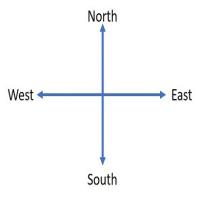Deadpool is between Falcon and Cyclops.

1. Antman, Batman, Cyclops, Deadpool, Electro, Falcon, and Gambit are standing near a wall and all of them are facing east.
2. Cyclops is on the immediate right of Deadpool.
3. Batman is at an extreme end and has Electro as his neighbor.
4. Gambit is between Electro and Falcon.
5. Deadpool is standing third from the south end.

Who is standing at the fourth position from north end?

a. Batman
b. Electro
c. Gambit
d. Falcon
 Answer  Explanation ANSWER: Falcon Explanation: Since all of them are facing East, the left and right should be taken into consideration accordingly.When a person is facing east, ‘Person’s Right’ will be towards South and ‘Person’s Left’ will be towards North.Cyclops is to the right of Deadpool.Deadpool is the third from south.So, Batman will be at extreme end from the north because it should have Electro as neighbor.Gambit is between Electro and Falcon.So, the sequence is –BatmanElectroGambitFalconDeadpoolCyclopsAntmanHere, Falcon is standing at fourth position from the north end.

8)   2, 5, 9, 19, 37, ?

a. 73
b. 75
c. 76
d. 78
 Answer  Explanation ANSWER: 75 Explanation: In this series, we have,2 x 2 + 1 = 55 x 2 - 1 = 99 x 2 + 1 = 1919 x 2 - 1 = 37So, the next term is 37 x 2 + 1 = 75

9)   In the following question, one term in the number series is wrong. Find the wrong term.

3, 2, 8, 12, 13, 24, 18, 32, 23, 42

a. 12
b. 13
c. 18
d. 24
 Answer  Explanation ANSWER: 24 Explanation: The given series is a combination of two series.I: 3 8 13 18 23II: 2 9 24 32 42In I, the pattern is + 5, in II the pattern is + 10The wrong number here is 24 in series II.The correct number should be: 12 + 10 = 22

10)   In the question below is given a statement followed by two courses of action numbered I and II. You have to assume everything in the statement to be true and based on the information given in the statement, decide which of the suggested courses of action logically follow(s) for pursuing.

(A) If only I follows
(B) If only II follows
(C) If either I or II follows
(D) If neither I nor II follow
(E) If both I and II follow.

Statement - The State Government has declared ‘Swine Flu’ as notifiable disease under the Epidemics Act.

Courses of Action - I. The Act should be implemented effective immediately.

II. The cases of punishment and stern actions should be propagated through media.

a. Only I follows
b. Only II follows
c. Either I or II follows
d. Neither I nor II follow
e. Both I and II follow
 Answer  Explanation ANSWER: Both I and II follow Explanation: The act is aimed at eradication of the disease.So, it needs to be promoted for the benefit of the people.Also, people must be aware of the stern actions if they do not cooperate. Here, both the courses follow.

11)   In the question below is given a statement followed by two courses of action numbered I and II. You have to assume everything in the statement to be true and based on the information given in the statement, decide which of the suggested courses of action logically follow(s) for pursuing.

(A) If only I follows
(B) If only II follows
(C) If either I or II follows
(D) If neither I nor II follow
(E) If both I and II follow.

Statement - Amandeep often insults his superiors in the office with his sarcastic remarks.

Courses of Action - I. He should be transferred to some other department.

II. The matter should be referred to the Board of Directors.

a. Only I follows
b. Only II follows
c. Either I or II follows
d. Neither I nor II follow
e. Both I and II follow
 Answer  Explanation ANSWER: Neither I nor II follow Explanation: In this case, transfer is not a good option as Amandeep may create the same problem in the other department too.Also, referring to Board of Directors for such problems is impractical. So, none of the courses follow.

12)   In the question below is given a statement followed by two conclusions numbered I and II.

(A) If only I follows
(B) If only II follows
(C) If either I or II follows
(D) If neither I nor II follows
(E) If both I and II follow.

Statement - Only good dancers are invited in the conference. Good dancers have a charming personality.

Conclusions - I. All invited dancers in the conference have charming personality.

II. Those dancers who do not have charming personality are not invited in the conference.

a. Only I follows
b. Only II follows
c. Either I or II follows
d. Neither I nor II follows
e. Both I and II follow
 Answer  Explanation ANSWER: Both I and II follow Explanation: The statement given here clearly states that good dancers have charming personality.According to given statement, those dancers who are not invited in the conference are not good dancers.Hence, I and II both follow.

13)   In the question below is given a statement followed by two conclusions numbered I and II.

(A) If only I follows
(B) If only II follows
(C) If either I or II follows
(D) If neither I nor II follows
(E) If both I and II follow.

Statement - In today’s world, no city is self-sufficient.

Conclusions - I. It is impossible to grow and produce all that a city needs.

II. Citizens have become very active.

a. Only I follows
b. Only II follows
c. Either I or II follows
d. Neither I nor II follows
e. Both I and II follow
 Answer  Explanation ANSWER: Only I follows Explanation: Here, Only I provides a suitable explanation to the given statement. So, only I follows.

• On a fine evening, before sunset, Rohit and Hannibal were talking facing each other.
• Hannibal's shadow was exactly to the right of Hannibal.
In which direction was Rohit facing?

a. East
b. West
c. North
d. South
 Answer  Explanation ANSWER: South Explanation: The sun sets in West. Hence, any shadow falls in the East before the sun sets. Hannibal's shadow was to the right of Hannibal.Hence, Rohit was facing towards South.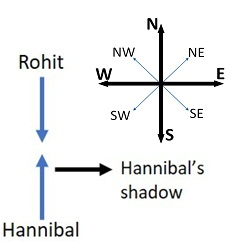• Rohit walked 50 m towards south, then he turned to his left and ran 40 m. He then turned to his left and walked 50 m.
• He again turned to his right and ran 30 m.
At what distance is he from the starting point and in which direction?

a. 70 m West
b. 75 m East
c. 75 m North
d. 70 m East
 Answer  Explanation ANSWER: 70 m East Explanation: Required distance = AE = 40 + 30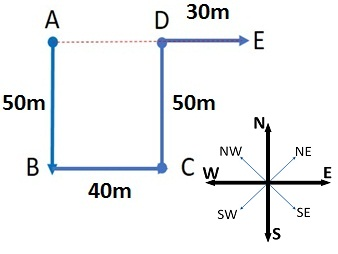16)   Pointing towards a girl, a man said to a woman, “Her mother is the only daughter of your mother.”

How is the girl related to that woman?

a. Sister
b. Daughter
c. Aunt
d. Wife
 Answer  Explanation ANSWER: Daughter Explanation: The only daughter of woman’s mother is woman herself.So, the girl is woman’s daughter i.e. the woman is the girl’s mother.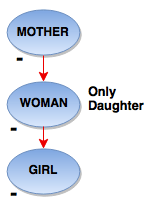There are six children playing football, namely, Z, Y, X, W, V and U.

1. Z and V are brothers.
2. U is the sister of V.
3. X is the only son of Z’s uncle.
4. Y and W are daughters of the brother of X’s father.

How is X related to U?

a. Cousin
b. Brother
c. Son
d. Uncle
 Answer  Explanation ANSWER: Cousin Explanation: U is V’s sister and hence Z’s sister.So, X is also son of U’s uncle and is, therefore, U’s cousin.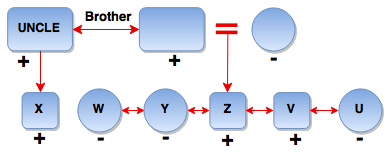18)   In question below is given a statement followed by two conclusions numbered I and II.

(A) If only I follows
(B) If only II follows
(C) If either I or II follows
(D) If neither I nor II follows
(E) If both I and II follow.

Statement I. All musicians are painters.
II. All dancers are painters.

Conclusion I. All dancers are musicians.
II. Some painters are not dancers.

a. Only I follows
b. Only II follows
c. Either I or II follows
d. Neither I nor II follows
e. Both I and II follow
 Answer  Explanation ANSWER: Neither I nor II follows Explanation: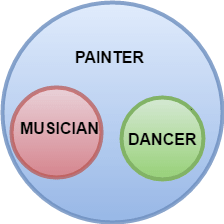19)   In the question below is given a statement followed by two conclusions numbered I and II.

(A) If only I follows
(B) If only II follows
(C) If either I or II follows
(D) If neither I nor II follows
(E) If both I and II follow.

Statement I. Every photograph is a portrait.
II. Every portrait is an artwork.

Conclusion I. Every photograph is an artwork.
II. Some artworks are portraits.

a. Only I follows
b. Only II follows
c. Either I or II follows
d. Neither I nor II follows
e. Both I and II follow
 Answer  Explanation ANSWER: Both I and II follow Explanation: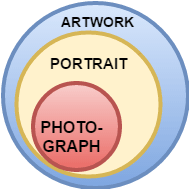20)   At what angle the hands of a clock are inclined at 40 minutes past 2?

a. 150°
b. 160°
c. 170°
d. 180°
 Answer  Explanation ANSWER: 160° Explanation: At 2 o’ clock, the hour hand is at 60° from the vertical. In 40 minutes,Hour hand = 60 + 40 x (0.5) = 80° (The hour hand moves at 0.5 dpm)Minute hand = 40 x (6) = 240° (The minute hand moves at 6 dpm)Difference or angle between the hands = 240 - 80 = 160°